# On the math of falling

#### Zacchaeus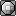Hello Smogon, I have seen that there are several rather intelligent members of this community, so I figured why not take my math question here?

In Algebra, for homework one night, I was given this question: A cliff diver dives off a cliff 40 feet above water. How long is the diver in the air?

The next day I asked my teacher "Is it possible to answer that question without knowing any other information like the diver's surface area or weight?" and she simply said "Yes" and did her little gravity equation.

Now I know that there are other things to take into account other than gravity when judging math regarding any sort of falling. This can easily be proven by taking two identical sheets of paper, then crunching one up into a small crumpled ball, and dropping them at the same time. They both weigh exactly the same, (which apparently isn't even a factor in the math of falling,) but hit the ground at completely different times.

So, what can you say about this?

#### Stathakis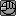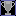the difference made by wind resistance is near negligible, so she probably just wants you to disregard it. just use the equations of kinematics and solve for the time spent in the air. you have enough known quantities to solve them.

#### VKCA

##### (Virtual Circus Kareoky Act)
Hello Smogon, I have seen that there are several rather intelligent members of this community, so I figured why not take my math question here?

In Algebra, for homework one night, I was given this question: A cliff diver dives off a cliff 40 feet above water. How long is the diver in the air?
This seems like more of a physics question
The next day I asked my teacher "Is it possible to answer that question without knowing any other information like the diver's surface area or weight?" and she simply said "Yes" and did her little gravity equation.

Now I know that there are other things to take into account other than gravity when judging math regarding any sort of falling. This can easily be proven by taking two identical sheets of paper, then crunching one up into a small crumpled ball, and dropping them at the same time. They both weigh exactly the same, (which apparently isn't even a factor in the math of falling,) but hit the ground at completely different times.

So, what can you say about this?
The reason for the phenomenon you describe is air resistance. Every item will accelerate at a rate of 9.8 m/s/s, if air resistance is negligible (which is the case for pretty much everything with the mass of greater than a kilogram falling a small enough distance to insure it does not reach it's terminal velocity), or if your in a vacuum.

#### ZacchaeusSo Fink, is my teacher wrong?

And to Stathakis, it just seems odd to me to ignore so many variables in a problem like that

#### Aura Guardian

If the variables are small enough, then they can be disregarded, especially for a non-precision problem like that. The diver may have jumped down a little to speed himself up, perhaps. So many factors that cancel out or total something small enough that it doesn't matter still don't matter even though they are many. Anyway, your teacher just wants to make sure you understand Algebra. The air resistance/whatever will come in later years and other classes. She told you what she needed to tell you for you to learn enough to get to the point where you can learn the complicated stuff.

#### ZacchaeusIf the variables are small enough, then they can be disregarded, especially for a non-precision problem like that. The diver may have jumped down a little to speed himself up, perhaps. So many factors that cancel out or total something small enough that it doesn't matter still don't matter even though they are many. Anyway, your teacher just wants to make sure you understand Algebra. The air resistance/whatever will come in later years and other classes. She told you what she needed to tell you for you to learn enough to get to the point where you can learn the complicated stuff.
Okay, thanks for the input. I obviously just haven't gotten to physics classes and whatnot so I was wondering just for my own purposes really, not so much for math class.

#### VKCA

##### (Virtual Circus Kareoky Act)
So Fink, is my teacher wrong?

And to Stathakis, it just seems odd to me to ignore so many variables in a problem like that
No, your teacher is correct. If you know the height of the cliff, and you know the speed at which the object is accelerating (9.8m/s/s) than you have all the info you need.

#### viol and bass

No, your teacher is correct. If you know the height of the cliff, and you know the speed at which the object is accelerating (9.8m/s/s) than you have all the info you need.
This is false. Technically air resistance and such are factors in this problem but seeing as this is a problem for algebra and not physics, it's most likely used as a setup for solving the problem in a specific way. As opposed to being focused on accuracy.

#### kiryu3000

Hello Smogon, I have seen that there are several rather intelligent members of this community, so I figured why not take my math question here?

In Algebra, for homework one night, I was given this question: A cliff diver dives off a cliff 40 feet above water. How long is the diver in the air?

The next day I asked my teacher "Is it possible to answer that question without knowing any other information like the diver's surface area or weight?" and she simply said "Yes" and did her little gravity equation.

Now I know that there are other things to take into account other than gravity when judging math regarding any sort of falling. This can easily be proven by taking two identical sheets of paper, then crunching one up into a small crumpled ball, and dropping them at the same time. They both weigh exactly the same, (which apparently isn't even a factor in the math of falling,) but hit the ground at completely different times.

So, what can you say about this?
I haven't been doing physics in a while and I may be a little rusty, but here goes:

Newton's Second Law states that any force acting on an object causes it to accelerate in the same direction. It is given in the equation F = mg = ma, where F is the force of gravity, m is the object's mass, g is the gravitational constant (9.8 N/kg), and a is the object's acceleration. As you can see in mg = ma, the mass cancels out on both sides, thus acceleration will always be 9.8m/s^2 on the surface of the earth and independent of mass.

On the subject of the paper, this is just pure guess, but I guess air resistance does have some effect here. Compare a crumpled piece of paper to a regular sheet of paper; the former has significantly less surface area than the latter. Air resistance is the result of an object colliding with air particles as it falls, and thus the lower the surface area, the lower the air resistance. Crumpled piece of paper is more dense than a sheet of paper, but I have run out of physics theories to explain that. I'm not even sure I'm right about the surface area idea, but it's just pure guess as I haven't been in physics class in a while.

#### darkie

##### .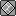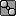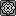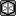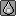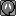On the subject of the paper, this is just pure guess, but I guess air resistance does have some effect here. Compare a crumpled piece of paper to a regular sheet of paper; the former has significantly less surface area than the latter. Air resistance is the result of an object colliding with air particles as it falls, and thus the lower the surface area, the lower the air resistance. Crumpled piece of paper is more dense than a sheet of paper, but I have run out of physics theories to explain that. I'm not even sure I'm right about the surface area idea, but it's just pure guess as I haven't been in physics class in a while.
Air resistance on a piece of paper makes it float. That IS adding additional variables. Try dropping two different objects heavy enough to not float at the same time. They will hit the ground at the same time if you drop them at the same time.

What grade are you in?

#### lati0s

Darkie, what do you mean by float? the paper is not floating on the air the same way a boat floats on water as it does reach the ground eventually.

#### kiryu3000

Air resistance on a piece of paper makes it float. That IS adding additional variables. Try dropping two different objects heavy enough to not float at the same time. They will hit the ground at the same time if you drop them at the same time.

What grade are you in?
Well, I never really did say that in confidence, but whatever. About the air resistance thing, it doesn't really make it "float", but rather opposes the force of gravity, which is why it descends to the ground slower than a dense object. I would say look into the center of mass, but as I've said I've gotten rusty in physics so there is a good chance I could be wrong.

#### Cathy

##### Banned deucer.
Just ignoring the air resistance issues here, I'd like to focus on a more basic aspect of physics that sheds some light on what other posters have taken for granted. Of course, if you're studied physics, this won't be anything new.

If the same force is applied to two different objects, they won't necessarily move with the same acceleration, because different objects have different resistances to acceleration. This resistance to acceleration is, of course, mass.

With that in mind, consider the acceleration one object, of electic charge q and mass m, will feel due to an electrostatic interaction with another object of electic charge Q, with a distance r joining their centres. Classically this force is given by the Coulomb force,

F = k q Q / r^2

And by Newton's second law,

a = (q / m) * (k Q / r^2)

where a denotes the acceleration of the first object, which is what we wanted to find. Notice how this depends both on its charge and its mass.

Gravity is very similar to electostatic interactions, and the strength of the interaction is based on what I will call an object's gravitational charge. So, we consider the same two objects above, but we consider the acceleration due to gravity rather than electrostatics: the only difference is we replace the q and Q by the object's gravitational charges, which I will denote x and X respectively, so the gravitational acceleration of the first object is given by the same formula as above except we replace the electric charges by the gravitational charges (and we select a different proportionality constant, G rather than k), giving:

a = (x / m) * (G X / r^2)

If we take r to be the radius of Earth, and X to be the gravitational charge of Earth, then the whole second bracketed part is a constant so I introduce g = G X / r^2 (which turns out to be the familiar 9.81... m/s^2), and write

a = (x / m) * g

So you can see the acceleration due to gravity of an object near the surface of Earth depends both on the object's mass (m) and its gravitational charge (x). But it turns out, experimentally, that an object's gravitational charge is exactly its mass, so x / m = 1 and a = g, which is constant.

This is pretty obvious to anybody who has studied any physics, but I wanted to highlight that the reason the acceleration due to gravity near the surface of Earth doesn't depend on any properties of the object being accelerated is that it turns out gravitational charge is mass. In that sense, gravitation is unlike any real force, and in some sense, it's not a force at all, but rather a "correction" that must be made in Newton's laws to account for working in a non-Euclidean geometry.

#### monkfish

##### formerly mfif an object is massive enough would it hit the earth faster due to exerting gravitational pull on the earth?

#### ultimifier

to OP. you are right, there is another force that acts on anything falling and it is known as drag. in simple terms it is the "friction" that the air puts on an object when it is falling. This drag force is also dependant on the velocity of the object sqaured.

so when falling a distance of 40 feet you are only going to be travelling around 15m/s when you hit the ground. 15^2 is 225. The terminal velocity for a human can be over 100m/s which is when the drag force is the same as gravity. 100^2 is 10000.

So at terminal velocty you have a force of 10000*C (a random constant) acting on you and when falling from 40 feet you have a force of 225*C acting on you.

As you can see the force of drag is very small until you get to high speeds which is why it can be neglected in a question like yours.

and also including drag requires advanced math that I didnt learn until first year university, so theres the REAL reason why your teacher doesnt want you to know about this drag, chances are they couldnt do the question themselves.

#### Cathy

##### Banned deucer.
if an object is massive enough would it hit the earth faster due to exerting gravitational pull on the earth?
Keep in mind, when we say the acceleration due to gravity near the surface of Earth is constant, the assumption is that the distance between the object and the surface of Earth is negligible compared to the radius of Earth. If we throw away that assumption, then the acceleration isn't constant, so different objects will take different times to fall the same distance to Earth.

However, the math involved here is quite complicated, and it's easier to just approximate it numerically. Using a very simple script and gnuplot, you can picture this graphically.

PHP:
``````<?php
\$mass = array(0.001, 1000); // set m and M here
\$separation = 100;
\$G = 0.00001;

\$x = array(0, \$separation);
\$v = array(0, 0);

\$t = 0;
while ((\$r = (\$x - \$x)) > 0) {
echo "\$t     \$r\n";
++\$t;
\$a0 = \$G * \$mass / (\$r * \$r);
\$a1 = \$G * \$mass / (\$r * \$r);
\$v += \$a0;
\$v -= \$a1;
\$x += \$v;
\$x += \$v;
}
?>``````The units here are arbitrary.

As you can see, it takes much longer for the two masses to converge (separation = 0) when one of them is a million times less massive than the other one.

#### MrIndigo

Physics is all about making approximations and ignoring variables; you just have to show that the contribution is very small compared to the other considerations.

For most mechanics, the friction between an object with density much greater than that of the air is several orders of magnitude smaller than the acceleration due to gravity, so you can take it as g=-10ms and get pretty accurate.

#### cantab

In Algebra, for homework one night, I was given this question: A cliff diver dives off a cliff 40 feet above water. How long is the diver in the air?

The next day I asked my teacher "Is it possible to answer that question without knowing any other information like the diver's surface area or weight?" and she simply said "Yes" and did her little gravity equation.
The question seems ill-posed. It fails to suggest the assumptions of neglecting air resistance, and that the diver's initial jump is horizontal. It doesn't even say "making reasonable assumptions". Thus, it is not possible to get an exact answer to the question as originally stated, nor is it even possible to get an answer with defined errors and confidence limits.

For most mechanics, the friction between an object with density much greater than that of the air is several orders of magnitude smaller than the acceleration due to gravity, so you can take it as g=-10ms and get pretty accurate.
For a 40 foot cliff, yes. For a 2,500 foot cliff (for example if you jumped off the cliff next to Angel Falls), you'd be way off if you neglected air resistance.

#### darkie

##### .Darkie, what do you mean by float? the paper is not floating on the air the same way a boat floats on water as it does reach the ground eventually.
I'm not using any official definition of "float" lol. I'm just saying that the sheet of paper seems to float.

#### WaterBomb

##### Two kids no brane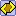Moderator
The answer is four hours, because the diver landed on top of a low flying plane that was on its way to BWI.

Sheesh you people are dumb.

#### tup

The answer is four hours, because the diver landed on top of a low flying plane that was on its way to BWI.

Sheesh you people are dumb.
uh your avatar has a cat.
Sorry for the off topic post by the way.

#### tape

##### i woke up in a new bugatti
In HS Physics, isn't it always assumed it's in a vacuum unless stated otherwise? Calculing something as drag and stuff seems a bit over the top.

I don't see what's the big fuss about this.

#### WaterBomb

##### Two kids no braneModerator
uh your avatar has a cat.
Sorry for the off topic post by the way.
And your avatar has the Dark Side of the Moon cover with something in the middle (is that the water beaver thingy from DP?)

#### N-Man

Just don't fall.

And if you do, breakfall and leave your calculator where it is.

#### ZacchaeusSorry for not posting in here for a while, I just really don't have the requisite knowledge to understand all this physics. Maybe if I come back and read this thread in a few years I'll understand it. Thanks for all the input though